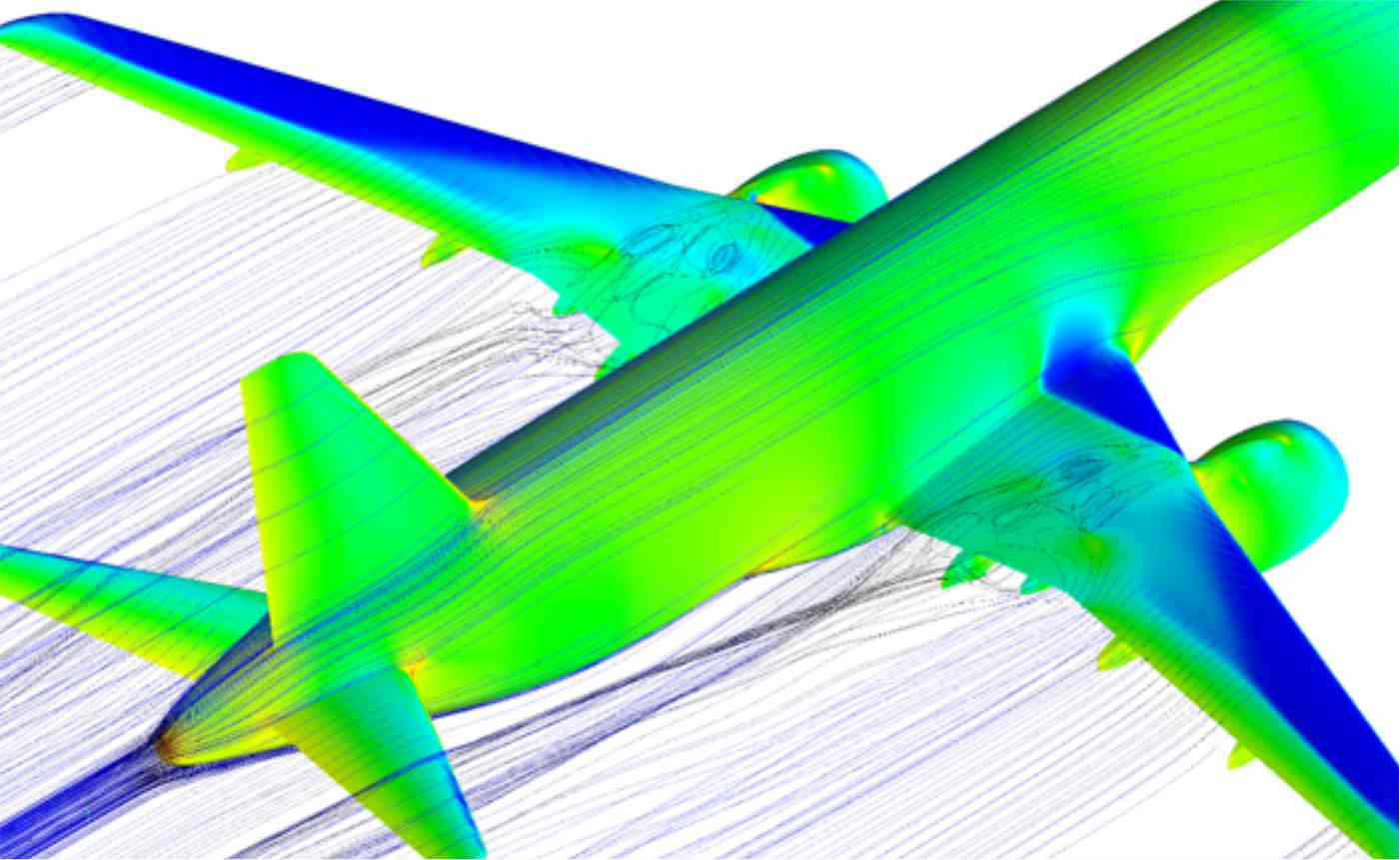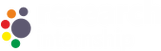0(0)

Course on Computational Fluid Dynamics

• Course level: Beginner
• Categories Mechanical
• Duration 40h
• Total Enrolled 0
• Last Update March 15, 2020

This course imparts the knowledge of how to formulate the governing differential equations of fluid dynamics and understand the mathematical characteristics of partial differential equations and then to solve Euler’s and Navier-Stokes equations of motion. Identify and implement numerical techniques for solving the space and time integration of partial differential equations.

Description

Computational Fluid Dynamics is essentially a branch of continuum mechanics which deals with numerical simulation of fluid flow and heat transfer problems.Mathematical modelling of continuum problems leads to a set of differentials, integral or integro-differential equations. Exact analytical solution of such equations will be limited to problems in simple geometries. Since most of the problems of interest based on industrial applications are complex problems, an approximate numerical solution is sought. In the context of mechanics, the science and practice of arriving an approximate numerical solution using digital computers is termed computational mechanics. When this is applied to thermo-fluid problems, the approach is commonly known as Computational Fluid Dynamics (CFD)which deals with approximate numerical techniques to solve space and time integral governing partial differential equations which are based on the fundamental conservation laws of physics, namely mass, momentum and energy conservation. This course includes the methodology for conversion of the governing equations for a continuum medium into a set of discrete algebraic equations using a process called discretization and then use the concepts of accuracy, stability, consistency of numerical methods for solving  the governing equations and arrive at the numerical solution to desired level of accuracy of the discrete equations by using a high speed digital computer.

What Will I Learn?

• Understand the fundamental of conservation laws of physics i.e. mass, momentum and energy conservation.
• Familiarity with formulating the mathematical modelling of continuum problems Understand mathematical characteristics of partial differential equations.
• Ability to frame the methodology for conversion of the governing equations for a continuum medium into a set of discrete algebraic equations.
• Identify and implement appropriate numerical techniques for solving the governing equations and arrive at the numerical solution to desired level of accuracy.

8 Lessons40h

Director, Research and Development

0 (0 ratings)

54 Courses

5 students10,000.00

Requirements

• The prerequisites required are fundamental knowledgein conservation laws of physics, namely mass, momentum and energy conservation. of measurements, Mathematical modelling of continuum problems,numerical techniques.

Target Audience

• Engineersworking in Mechanical, automotive, aerospace, railways, defence, nuclear energy, thermal power, wind, medical equipment, civil, electrical and electronics etc, and related Industry involving inthermo-fluid analysis can participate.
Virtual Engineering
# 28 (Old No 619/1), 2nd Floor
36th Cross, 2nd Block, Rajajinagar
Bangalore-560010 India
info@virtual-engineering.com